## Shah Function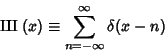(1)

where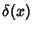is the Delta Function, so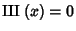for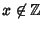(i.e.,not an Integer). The shah function obeys the identities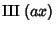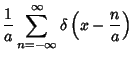(2)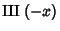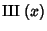(3)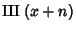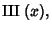(4)

for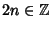(i.e.,a half-integer).

It is normalized so that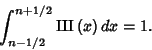(5)

The sampling property'' is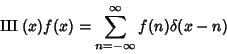(6)

and the replicating property'' is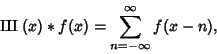(7)

where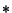denotes Convolution.